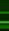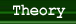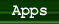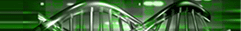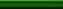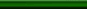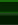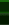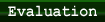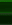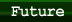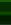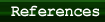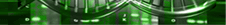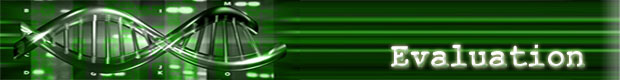### I. Benefits of DNA Computing

a. Performance rate
Performing millions of operations simultaneously allows the performance rate of DNA strands to increase exponentially. Adleman’s experiment was executed at 1,014 operations per second, a rate of 100 Teraflops (100 trillion floating point operations per second). The world’s fastest supercomputer runs at just 35.8 Teraflops (Srivastava).

b. Parallel processing
The massively parallel processing capabilities of DNA computers has the potential of speeding up large, but otherwise solvable, polynomial time problems requiring relatively few operations (Adams). For instance, a mix of 1,018 strands of DNA could operate at 10,000 times the speed of today’s advanced supercomputers (Parker).

c. Ability to hold tremendous amounts of info in very small spaces
Traditional storage media, such as videotapes, require 10^12 cubic nanometers of space to store a single bit of information; DNA molecules require just one cubic nanometer per bit (Parker). In other words, a single cubic centimeter of DNA holds more information than a trillion CDs (Johnson). This is because the data density of DNA molecules approaches 18 Mbits per inch, whereas today’s computer hard drives can only store less than 1/100,000 of this information in the same amount of space (Srivastava).

Summary
The DNA computer has clear advantages over conventional computers when applied to problems that can be divided into separate, non-sequential tasks. The reason is that DNA strands can hold so much data in memory and conduct multiple operations at once, thus solving decomposable problems much faster. On the other hand, non-decomposable problems, those that require many sequential operations are much more efficient on a conventional computer due to the length of time required to conduct the biochemical operations (Adams).

### II. Limitations

a. Requires exponential resource in terms of memory
Generating solution sets, even for some relatively simple problems, may require impractically large amounts of memory (Adams). Although DNA can store a trillion times more information than current storage media, the way in which the information is processed necessitates a massive amount of DNA if larger-scale problems are to be solved (Parker).

b. Accuracy
DNA synthesis is liable to errors, such as mismatching pairs, and is highly dependent on the accuracy of the enzymes involved (Parker). In addition, the chance of errors increases exponentially, limiting the number of operations you can do successively before the probability becomes greater than producing the correct result (Adams).

c. Resource-intensive
i.   Each stage of parallel operations requires time measured in hours or days, with extensive human or mechanical intervention between steps (Adams).

ii.  Since a set of DNA strands is tailored to a specific problem, a new set would have to be made for each new problem (Kiernan).

iii.   Algorithms can be executed in polynomial time due to the massive parallelism inherent in DNA computation, but they are limited in applicability to small instances of these problems because they require the generation of an unrestricted solution space. For example, the DNA encoding of all paths of a Traveling Salesman problem with 200 cities would weigh more than the earth (Miller).

Summary
Overall, many technological challenges remain before DNA computing can be widely used. New techniques must be developed to reduce the number of computational errors produced by unwanted chemical reactions with the DNA strands, and steps in processing DNA need to be eliminated, combined or accelerated (Kiernan).

### III. DNA vs. Silicon

As mentioned in previous sections, DNA performs parallel operations while conventional, silicon-based computers typically handle operations sequentially. A modern CPU basically repeats the same “fetch and execute (fetches an instruction and the appropriate data from main memory and executes it) cycle” over and over again. This process is repeated many, many times in a row, and really, really fast. Typically, increasing performance of silicon computing means faster clock cycles, placing emphasis on the speed of the CPU and not on the size of the memory. Oppositely, the power of DNA computing comes from its memory capacity and parallel processing. In other words, DNA loses its appeal if forced to behave sequentially.

Example 1:
In bacteria, DNA can be replicated at a rate of about 500 base pairs a second (1000 bits/sec). Biologically, this is quite fast, but very slow when compared to the speed of an average hard drive. However, when DNA operations work in parallel, the replication enzymes can start on the second replicated strand of DNA even before they're finished copying the first one. Basically, the number of DNA strands increases exponentially (2^n after n iterations), and with each additional strand, the data rate increases by 1000 bits/sec. So after 30 iterations it increases to 1000 Gbits/sec—this is beyond the sustained data rates of the fastest hard drives (Adams).

Example 2: Traveling Salesman Problem with more than 10 cities
With a conventional computer, one method would be to set up a search tree, measure each complete branch sequentially, and keep the shortest one. A very laborious method would be to generate all possible paths and then search the entire list! With this algorithm, generating the entire list of routes for a 20-city problem could theoretically take 45 million GBytes of memory. This would take a 100 MIPS (million instructions per second) computer two years just to generate all paths (assuming one instruction cycle to generate each city in every path). However, using DNA computing, this method becomes feasible because 10^15 is just a nanomole of material, a relatively small number for biochemistry. Also, routes no longer have to be searched through sequentially: operations can be done all in parallel (Adams).